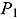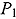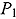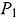# The Discontinuous Asymptotic Telegrapher’s Equation (P1) Approximation

Nuclear Science and Engineering / Volume 192 / Number 2 / November 2018 / Pages 189-207

Technical Paper / dx.doi.org/10.1080/00295639.2018.1499339

Modeling the propagation of radiative heat waves in optically thick material using a diffusive approximation is a well-known problem. In optically thin material, classic methods, such as classic diffusion or classic, yield the wrong heat wave propagation behavior, and higher-order approximation might be required, making the solution more difficult to obtain. The asymptoticapproximation [Heizler, Nucl. Sci. Eng., Vol. 166, p. 17 (2010)] yields the correct particle velocity but fails to model the correct behavior in highly anisotropic media, such as problems that involve a sharp boundary between media or strong sources. However, the solution for the two-region Milne problem of two adjacent half-spaces divided by a sharp boundary yields a discontinuity in the asymptotic solutions that makes it possible to solve steady-state problems, especially in neutronics. In this work we expand the time-dependent asymptoticapproximation to a highly anisotropic medium using the discontinuity jump conditions of the energy density, yielding a modified discontinuousequation in general geometry. We introduce numerical solutions for two fundamental benchmarks in plane symmetry. The results thus obtained are more accurate than those attained by other methods, such as Flux Limiters or Variable Eddington Factors.